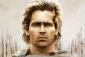# Find the value of x

2 posts / 0 new
AlexanderFind the value of x

(x + y) = 5

fitzmerl duron$x = 5 - y$

The equation for $y$ is unknown.

Thanks!

• Mathematics inside the configured delimiters is rendered by MathJax. The default math delimiters are $$...$$ and $...$ for displayed mathematics, and $...$ and $...$ for in-line mathematics.